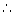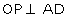ICSE Class 9 Circle Solution New Pattern

## ICSE Class 9 Circle Solution New Pattern By Clarify Knowledge

ICSE Class 9 Circle Solution New Pattern 2022

## Chapter 17 - Circle Exercise Ex. 17(A)

Question 1

A chord of length 6 cm is drawn in a circle of radius 5 cm. Calculate its distance from the centre of the circle.Solution 1

Let AB be the chord and O be the centre of the circle.

Let OC be the perpendicular drawn from O to AB.

We know, that the perpendicular to a chord, from the centre of a circle, bisects the chord.AC = CB = 3 cm

Question 2

A chord of length 8 cm is drawn at a distance of 3 cm from the centre of the circle. Calculate the radius of the circle.Solution 2

Let AB be the chord and O be the centre of the circle.

Let OC be the perpendicular drawn from O to AB.

We know, that the perpendicular to a chord, from the centre of a circle, bisects the chord.

Hence, radius of the circle is 5 cm.Question 3

The radius of a circle is 17.0 cm and the length of perpendicular drawn from its centre to a chord is 8.0 cm. Calculate the length of the chord.Solution 3

Let AB be the chord and O be the centre of the circle.

Let OC be the perpendicular drawn from O to AB.

We know, that the perpendicular to a chord, from the centre of a circle, bisects the chord.AC = CB

Question 4

A chord of length 24 cm is at a distance of 5 cm from the centre of the circle. Find the length of the chord of the same circle which is at a distance of 12 cm from the centre.Solution 4

Let AB be the chord of length 24 cm and O be the centre of the circle.

Let OC be the perpendicular drawn from O to AB.

We know, that the perpendicular to a chord, from the centre of a circle, bisects the chord.AC = CB = 12 cm

Question 5

In the following figure, AD is a straight line,and O is the centre of both the circles. If OA = 34cm, OB = 20 cm and OP = 16 cm; find the length of AB.

Solution 5

For the inner circle, BC is a chord and.

We know that the perpendicular to a chord, from the centre of a circle, bisects the chord.BP = PC

For the outer circle, AD is the chord and.

We know that the perpendicular to a chord, from the centre of a circle, bisects the chord.AP = PD

By Pythagoras Theorem,

OA2 = OP2 + AP2

=> AP2 = (34)2 - (16)= 900

=> AP = 30 cm

AB = AP - BP = 30 - 12 = 18 cmQuestion 6

In a circle of radius 17 cm, two parallel chords of lengths 30 cm and 16 cm are drawn. Find the distance between the chords, if both the chords are:

(i) on the opposite sides of the centre;

(ii) on the same side of the centre.Solution 6

Let O be the centre of the circle and AB and CD be the two parallel chords of length 30 cm and 16 cm respectively.

Drop OE and OF perpendicular on AB and CD from the centre O.

Question 7

Two parallel chords are drawn in a circle of diameter 30.0 cm. The length of one chord is 24.0 cm and the distance between the two chords is 21.0 cm; find the length of another chord.Solution 7

Since the distance between the chords is greater than the radius of the circle (15 cm), so the chords will be on the opposite sides of the centre.

Let O be the centre of the circle and AB and CD be the two parallel chords such that AB = 24 cm.

Let length of CD be 2x cm.

Drop OE and OF perpendicular on AB and CD from the centre O.

Question 8

A chord CD of a circle whose centre is O, is bisected at P by a diameter AB. Given OA = OB = 15 cm and OP = 9 cm. Calculate the lengths of:

(i) CD ; (ii) AD ; (iii) CB.

Solution 8

Question 9

The figure given below shows a circle with centre O in which diameter AB bisects the chord CD at point E. If CE = ED = 8 cm and EB = 4 cm, find the radius of the circle.

Solution 9

Let the radius of the circle be r cm.

Question 10

In the given figure, O is the centre of the circle. AB and CD are two chords of the circle. OM is perpendicular to AB and ON is perpendicular to CD. AB = 24 cm, OM = 5 cm, ON = 12 cm, Find the :

(ii) length of chord CD

Solution 10

## Chapter 17 - Circle Exercise Ex. 17(B)

Question 1

The figure shows two concentric circles and AD is a chord of larger circle. Prove that: AB = CD.

Solution 1

Question 2

A straight line is drawn cutting two equal circles and passing through the mid-point M of the line joining their centres O and O'. Prove that the chords AB and CD, which are intercepted by the two circles, are equal.

Solution 2

Question 3

M and N are the mid-points of two equal chords AB and CD respectively of a circle with centre O. Prove that:

Solution 3

Question 4

In the following figure; P and Q are the points of intersection of two circles with centres O and O'. If straight lines APB and CQD are parallel to OO'; prove that:

Solution 4

Drop OM and O'N perpendicular on AB and OM' and O'N' perpendicular on CD.

Question 5

Two equal chords AB and CD of a circle with centre O, intersect each other at point P inside the circle. Prove that:

(i) AP = CP ; (ii) BP = DP

Solution 5

Drop OM and ON perpendicular on AB and CD.

Join OP, OB and OD.

Question 6

In the following figure, OABC is a square. A circle is drawn with O as centre which meets OC at P and OA at Q. Prove that:

Solution 6

Question 7

The length of the common chord of two intersecting circles is 30 cm. If the diameters of these two circles be 50 cm and 34 cm, calculate the distance between their centres.Solution 7

Question 8

The line joining the midpoints of two chords of a circle passes through its centre. Prove that the chords are parallel.Solution 8

Question 9

In the following figure, the line ABCD is perpendicular to PQ; where P and Q are the centres of the circles. Show that:

(i) AB = CD ;

(ii) AC = BD.

Solution 9

Question 10

AB and CD are two equal chords of a circle with centre O which intersect each other at right angle at point P. If OMAB and ONCD; show that OMPN is a square.Solution 10

## Chapter 17 - Circle Exercise Ex. 17(C)

Question 1

In the given figure, an equilateral triangle ABC is inscribed in a circle with centre O.

Find: (i) ∠BOC (ii) ∠OBC

Solution 1

Question 2

In the given figure, a square is inscribed in a circle with centre O. Find:

(i) ∠BOC

(ii) ∠OCB

(iii) ∠COD

(iv) ∠BOD

Is BD a diameter of the circle?

Solution 2

Question 3

In the given figure, AB is a side of regular pentagon and BC is a side of regular hexagon.

(i) ∠AOB

(ii) ∠BOC

(iii) ∠AOC

(iv) ∠OBA

(v) ∠OBC

(vi) ∠ABC

Solution 3

Question 4

In the given figure, arc AB and arc BC are equal in length.

If ∠AOB = 48°, find:

(i) ∠BOC

(ii) ∠OBC

(iii) ∠AOC

(iv) ∠OAC

Solution 4

Question 5

In the given figure, the lengths of arcs AB and BC are in the ratio 3:2.

If ∠AOB = 96°, find:

(i) ∠BOC

(ii) ∠ABC

Solution 5

Question 6

In the given figure, AB = BC = DC and ∠AOB = 50°.

(i) ∠AOC

(ii) ∠AOD

(iii) ∠BOD

(iv) ∠OAC

(v) ∠ODA

Solution 6

Question 7

In the given figure, AB is a side of a regular hexagon and AC is a side of a regular eight sided polygon. Find:

(i) ∠AOB

(ii) ∠AOC

(iii) ∠BOC

(iv) ∠OBC

Solution 7

Question 8

In the given figure, O is the centre of the circle and the length of arc AB is twice the is twice the length of arc BC.

If ∠AOB = 100°, find:

(i) ∠BOC

(ii) ∠OAC

Solution 8

## Chapter 17 - Circle Exercise Ex. 17(D)

Question 1

The radius of a circle is 13 cm and the length of one of its chords is 24 cm. Find the distance of the chord from the centre.Solution 1

Question 2

Prove that equal chords of congruent circles subtend equal angles at their centre.Solution 2

Question 3

Draw two circles of different radii. How many points these circles can have in common? What is the maximum number of common points?Solution 3

So, the circle can have 0, 1 or 2 points in common.

The maximum number of common points is 2. Question 4

Suppose you are given a circle. Describe a method by which you can find the centre of this circle.Solution 4

Question 5

Given two equal chords AB and CD of a circle with centre O, intersecting each other at point P. Prove that:

(i) AP = CP

(ii) BP = DP

Solution 5

Question 6

In a circle of radius 10 cm, AB and CD are two parallel chords of lengths 16 cm and 12 cm respectively. Calculate the distance between the chords, if they are on:

(i) the same side of the centre.

(ii) the opposite sides of the centre.Solution 6

Question 7

In the given figure, O is the centre of the circle with radius 20 cm and OD is perpendicular to AB.

If AB = 32 cm, find the length of CD

Solution 7

Question 8

In the given figure, AB and CD are two equal chords of a circle, with centre O.

If P is the mid-point of chord AB, Q is the mid-point of chord CD and ∠POQ = 150°, find ∠APQ.

Solution 8

Question 9

In the given figure, AOC is the diameter of the circle, with centre O.

If arc AXB is half of arc BYC, find ∠BOC.Solution 9

Question 10

Solution 10

error: Content is protected !!